Courses

# Test: Oxidation Reduction And Disproportionation Reactions

## 23 Questions MCQ Test Chemistry for JEE | Test: Oxidation Reduction And Disproportionation Reactions

Description
This mock test of Test: Oxidation Reduction And Disproportionation Reactions for JEE helps you for every JEE entrance exam. This contains 23 Multiple Choice Questions for JEE Test: Oxidation Reduction And Disproportionation Reactions (mcq) to study with solutions a complete question bank. The solved questions answers in this Test: Oxidation Reduction And Disproportionation Reactions quiz give you a good mix of easy questions and tough questions. JEE students definitely take this Test: Oxidation Reduction And Disproportionation Reactions exercise for a better result in the exam. You can find other Test: Oxidation Reduction And Disproportionation Reactions extra questions, long questions & short questions for JEE on EduRev as well by searching above.
QUESTION: 1

### Direction (Q. Nos. 1-18) This section contains 18 multiple choice questions. Each question has four choices (a), (b), (c) and (d), out of which ONLY ONE option is correct. Q. Oxidation can be defined as the terms I. gain of electron and hydrogen II. gain of oxygen and loss of electron III. increase in oxidation number IV. decrease in oxidation number Select the correct terms

Solution: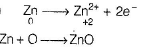Oxidation number increases, oxygen gained and loss of electrons is oxidation.

QUESTION: 2

### Reduction is defined in terms of I. electronation and hydrogenation II. deelectronation and gain of oxygen III. increase in oxidation number IV. decrease in oxidation number Select the correct terms

Solution:

Gain of hydrogen , electron and decrease in oxidation number is reduction

QUESTION: 3

### In balancing the half-reaction, CN- → CNO- The number of electrons that must be added is

Solution:

CN→ CNO-
4+ x = -1      4+ x-2 = -1
∴ x = -5    ∴ x = -3
Oxidation number increases. This is oxidation.

CN→ CNO- + 2e-

QUESTION: 4

Select the set of compounds with oxidation-reduction duality.

Solution:

Com pounds having oxidising and reducing nature in given reaction are said to have oxidation-reduction duality. Such compounds are said to undergo disproportionation reaction.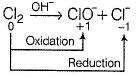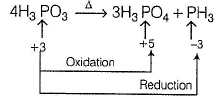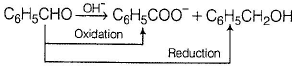(This is called Cannizzaro reaction.)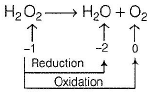Note Such compounds have O.N. of the affected atoms intermediate of oxidation part and reduction part

QUESTION: 5

In the following balanced reaction,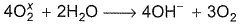Solution:

If balanced, then to balance charge
x = - 1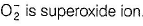QUESTION: 6

I reduces IO3- and I2 and itself oxidised to I2 in acidic medium. Thus, final reaction is

Solution: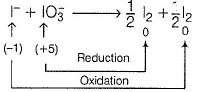To balance oxidation number, cross-multiply by change in oxidation number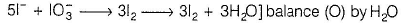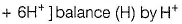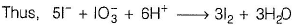QUESTION: 7

H2O can be oxidised to

Solution: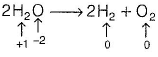H is reduced to H2 and O is oxidised to O2.

QUESTION: 8

Consider the following reactions,

I. Zn + dil. H2SO4 → ZnSO4 + H2
II. Zn + conc. H2SO4 → ZnSO4+ SO2 + H2O

Oxidising agents in I and II are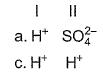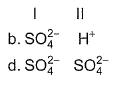Solution: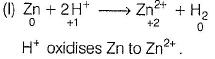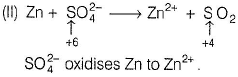QUESTION: 9

In the following reactions except in one, oxygen is the reducing agent. Exceptional reaction is

Solution: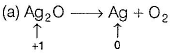Ag+ is reduced to Ag by oxygen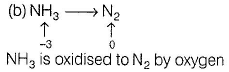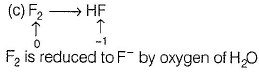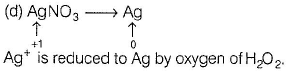Thus, except in (b), oxygen behaves as reducing agent.

QUESTION: 10

In the following reaction,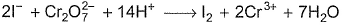Which ions are not balanced?

Solution: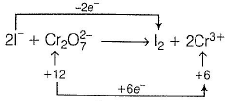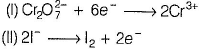To balance electron  (II)  is to be multiplied by (3). Thus,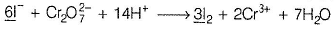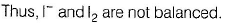QUESTION: 11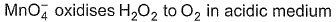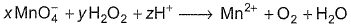Coefficient x, y and z are respectively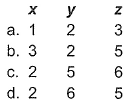Solution: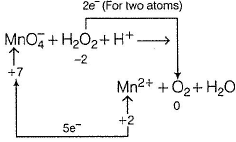Cross -multiply by change in oxidation number and balance H by H+ ions.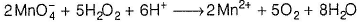Thus, x = 2, y = 5 and z = 6

QUESTION: 12

Which of the following species does not show disproportionation reaction?

Solution:

Maximum oxidation number of Cl = + 7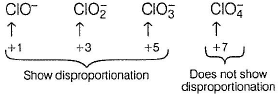Thus, species with + 7 oxidation number can be reduced but not oxidised.

QUESTION: 13

Which is the intramolecular oxidation-reduction reaction?

Solution: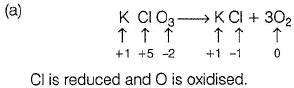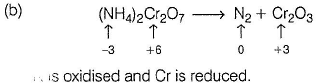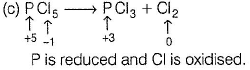QUESTION: 14

Consider the following experimental facts,

I. When Cl2 gas is passed into Kl solution containing CHCI3, violet colour appears in CHCI3 layer.
II. When Cl2 gas is passed into KBr solution containing CHCI3, orange colour appears in CHCI3 layer.
III. When Cl2 gas is passed into a solution containing KBr, Kl and KCI, containing CHCI3, violet colour appears in CHCI3 layer.

Select the correct experimental facts.

Solution:

Oxidising power of F2 > CI2 > Br2 > l2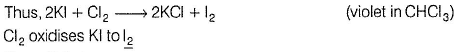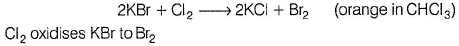Thus, (II) is true.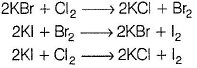In experiment (III), orange colour of Br2 does not appear, as it also oxidises Kl to l2. Hence , only violet colour appears in CHCI3 layer. Thus, (III) is also true.

QUESTION: 15

Based on the following reaction,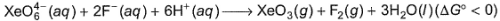It can be concluded that

Solution: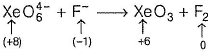Since, ΔG ° < 0, hence it is spontaneous in forward direction . Oxidation number of Xe decreases hence, it is an oxidising agent and oxidation number of F increases, hence it is a reducing agent.

QUESTION: 16

For the redox reaction,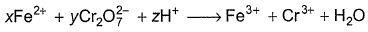x, y and z are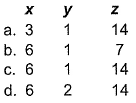Solution: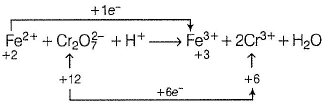To balance a redox reaction , balance effected atoms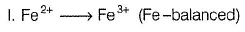II. Take oxidation number of two Cr-atom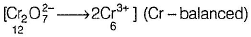Take total (oxidation number) of each atom (oxidised, re duced ) cross - multiply by change in oxidation number and add
I x 6 + II x 1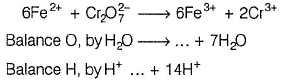Thus, x = 6
y = 1
z = 14

QUESTION: 17

In the following conversion of chromite into soluble chromium salt
4Fe(CrO2)2+ 8Na2CO3 + 7O2 --> 2Fe2O3 + 8Na2CrO4 + 8CO2

There is

Solution: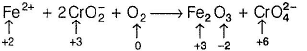Fe 2+ and Cr3+ are oxidised (increase in oxidation number)
O2, is reduced .

QUESTION: 18

In which of the following reactions H2O2 acts as a reducing agent?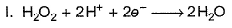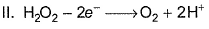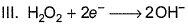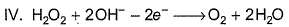Solution:

A species which is oxidised (deelectronation) is a reducing agent.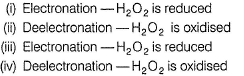Thus, (ii) and (iv) represent reducing behaviour of H2O2.

*Multiple options can be correct
QUESTION: 19

Consider the following reaction,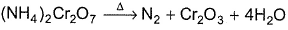Solution: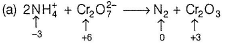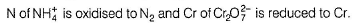Both elements are present in the same substance . Such reaction is called intramolecular redox reaction.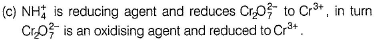*Multiple options can be correct
QUESTION: 20

Following reaction,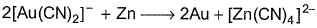is an example of

Solution:

(a) Zn is oxidised to Zn2+ in complex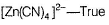(b) Au+ is reduced to Au — True
(c) Au (s) is displaced
(d) Since, it is a spontaneous reaction ΔG° < 0

*Multiple options can be correct
QUESTION: 21

Select the correct statement(s).

Solution: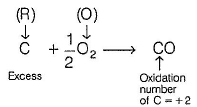Carbon is reducing agent (R) and oxygen is oxidising agent (O). CO with oxidation number of C (= + 2) is formed.
(R) is inexcess hence, product of lower (oxidation number) is formed.
Thus, (a) is correct.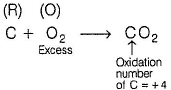(O) is in excess hence product of higher oxidation number is formed. Thus, (b) is also correct.

*Multiple options can be correct
QUESTION: 22

For the reaction,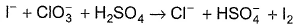The correct statement(s) in the balanced equation is/are

[JEE Advanced 2014]

Solution: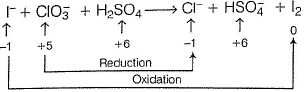To balance a redox reaction,
Step I Balance effected atoms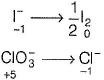Step II Cross-multiply by change in oxidation number and add H2O to balance 0 (on RHS)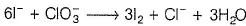Add H+ to balance H (on LHS)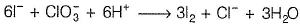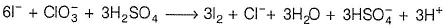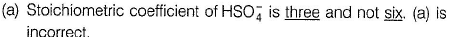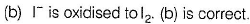(c) No effect on S. (c) is incorrect.
(d) H2O is one of the products, (d) is correct.

*Multiple options can be correct
QUESTION: 23

Identify intramolecular oxidation-reduction reaction.

Solution: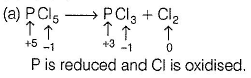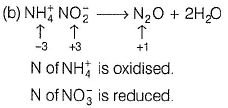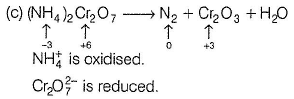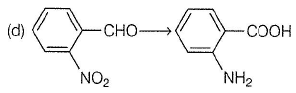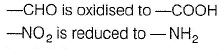In all of the above reactions, oxidised and reduced part are in same species, hence, intramolecular redox reactions.